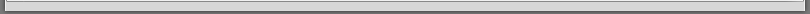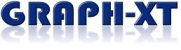With GRAPH-XT for HTBasic you create stunning, high quality 2D and 3D diagrams from your measurement data with just a few lines of code. Buy GRAPH-XT today and turn your data into eye-opening charts !

Don't mess with VIEWPORTs, WINDOWs or CLIPping values anymore, simply collect your data and GRAPH-XT will create presentation-quality  plots  with  automatic  scaling.  While  geared  towards  scientists,  engineers,  and  other  technical  fields, GRAPH-XT's straightforward interface will appeal to anybody interested in creating X-Y plots and/or contour plots of 3D data.

HTBasic's graphic capabilities now show it's age in modern applications. Do you need better quality, special diagram types or want to display 3D data? Then we might be able to help you!

Check out GRAPH-XT today using the fully featured demo version free of charge !

 Examples Would one be able to create the diagrams below using traditional HTBasic graphics commands only? Click on the pictures below for full size view.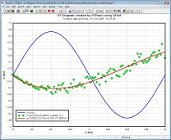XY Diagram This shows a simple XY diagram with 3 curves and the number format 'fractions of PI' for the x axis. Curve 2 is a scatter plot (only data points are shown with no line segments between them). Curve 3 is a curve fit of curve 2 using the built-in curve fitting functions of DPlot.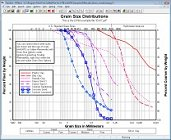Grain Size Distribution Plot Grain size distribution plots are typically used in sieve analyses.Multiple Diagrams This example shows four independant diagrams on one HTBasic screen.Bitmap Import Filter Demonstration of the bitmap import filter. A bitmap has been imported and shown as a 3D contour plot, shown in high colour mode.Another 3D Contour Plot 3D contour plot of the equation 'z=4-(SIN(SQR(x^2+y^2)-3)+SQR(x^2+y^2)-3)', shown in high colour mode.SRTM Elevation Data Import Filter 3D contour plot of an imported SRTM data file, shown in high colour mode.Three Dependant Diagrams Shows an imported wav file, an FFT and the histogram derived from the wav file data.ArcView Shape File Import Filter Map of the world (without Antarctica) created from imported ArcView shape files.2D Contour Plot 2D L-shaped contour plot of an electrode.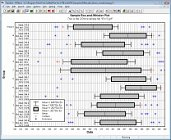Box-and-Whisker Plot In descriptive statistics, a box-and-whisker plot is a convenient way of graphically depicting groups of numerical data through their five-number summaries (the smallest observation, lower quartile (Q1), median (Q2), upper quartile (Q3), and largest observation).Triangle Plot A triangle plot (also known as a ternary plot), is a graph of 3 variables. It is most often used in geologic studies to show the relative compositions of soils and rocks, but it can be more generally applied to any system of three variables.Tripartite Plot A tripartite plot (also known as "four coordinate paper") consists of a logarithmic scale on both the X and Y axes and two additional logarithmic scales that are derived from the X and Y axes by some fixed relationship. It is typically used in vibration analyses.

 GRAPH-XT is another product developed by Tech Soft "made in Germany".
 System Requirements    Operating system: Windows 2000 / XP / 2003 / Vista / 7 / 8 / 10    HTBasic for Windows Release 9.2 or higher

Ordering Information

 Order Code Description H-TP0010 GRAPH-XT H-TP1010 GRAPH-XT Update (Proof of previous version ownership required)